# RS Aggarwal Class 9 Solutions Chapter 12 - Geometrical Constructions

## RS Aggarwal Class 9 Chapter 12 - Geometrical Constructions Solutions Free PDF

Geometrical constructions teach students about the step-by-step construction procedure for different shapes. Some of the various tools that we would need in order to construct the figures are a ruler, compass, divider, set-squares and a protractor. This kind of construction is considered as the pure form of construction without any numbers and students can gain proficiency in drawing different shapes, angles and lines.

Some of the different constructions that students need to be well versed in for the final examinations are:

1. The parallel line through a point
2. Congruent Angle
3. Equilateral Triangle
4. 45,60,90 and 120-degree angles
5. Center of a circle

## Download PDF of RS Aggarwal Class 9 Solutions Chapter 12 – Geometrical Constructions

The solutions we have provided here are highly effective for exam preparation. So, just refering to the RS Aggarwal solutions of Class 9 will help in exam preparation in the right channel. Refer to these RS Aggarwal Class 9 solutions whenever you have any doubt or get stuck solving exercise questions.

Q.1: Draw a line segment AB = 5 cm and draw its perpendicular bisector.

Sol:

Steps of Construction:

(i) Draw a line segment AB= 5cm

(ii) With A as center and radius equal to more than half of AB, draw two arcs, one above AB and the other below AB.

(iii) With B as a center and the same radius draw two arcs which cut the previously drawn arcs at C and D.

(iv) Join CD, intersecting AB at point P.

Therefore, CD is the perpendicular bisector of AB at the point P.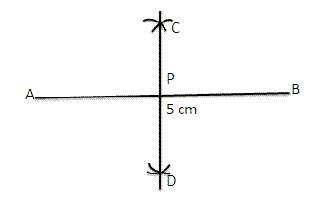Q.2: Draw an angle of 450 using scale and compass only. Draw the bisector of this angle.

Sol:

Steps of Construction:

(i) Draw a line segment OA.

(ii) At A, draw AOE=90$\angle AOE=90^{\circ}$, using rulers and compass.

(iii) With B as center and radius more than half of BD, draw an arc.

(iv) With D as a center and same radius draw another arc which cuts the previous arc at F.

(v) Join OF. AOF=45$\angle AOF=45^{\circ}$

(vi) Now with center B and radius more than half of BC, draw an arc.

(vii) With center C and same radius draw another arc which cuts the previously drawn arc at X.

(viii) Join OX.

Therefore, OX is the bisector of AOF$\angle AOF$.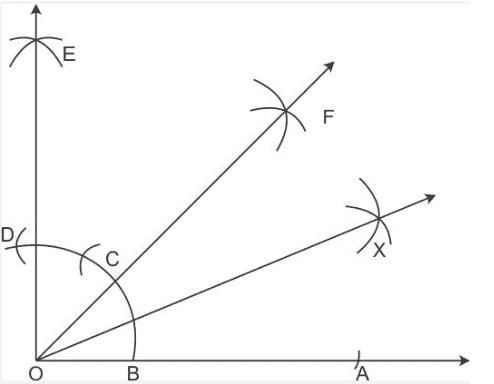Q.3: Draw an angle of 900 and draw its bisector.

Sol:

Steps of construction:

(i) Draw a line segment OA.

(ii) With O as the center and any suitable radius draw an arc, cutting OA at B.

(iii) With B as the center and the same radius cut the previously drawn arc at C.

(iv) With C as the center and the same radius cut the arc at D.

(v) With C as the center and the radius more than half CD draw an arc.

(vi) With D as the center and the same radius draw another arc which cuts the previous arc at E.

(vii) Join E, So AOE=90$\angle AOE=90^{\circ}$

(viii) Now with B as center and radius more than half of CB draw an arc.

(ix) With C as the center and same radius draw an arc which cuts the previous at F.

(x) Join OF. Therefore, it is the bisector of the right AOE$\angle AOE$.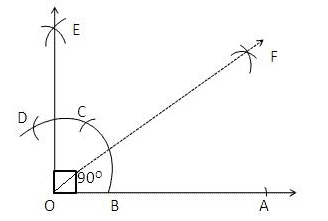Q.4: Construct an equilateral triangle each of whose sides measures 5 cm.

Sol:

Steps of construction:

(i) Draw a line segment BC= 5cm

(ii) With B as center and radius equal to BC draw an arc.

(iii) With C as the center and the same radius draw another arc which cuts the previous arc at A.

(iv) Join AB and AC.

Then ABC$\bigtriangleup ABC$ is the required equilateral triangle.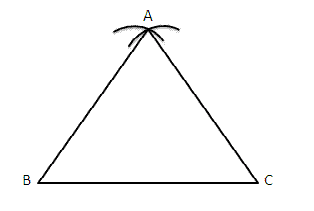Q.5: Construct an equilateral triangle each of whose altitudes measures 5.4 cm

Sol:

Steps of construction:

(i) Draw a line XY.

(ii) Mark any point P on it.

(iii) From P, draw PQXY.$PQ\perp XY.$

(iv) From P, set off PA= 5.4 cm cutting PQ at A.

(v) Construct PAC=30$\angle PAC=30^{\circ}$, meeting XY at B and C respectively.

Therefore, ABC$\bigtriangleup ABC$ is required equilateral triangle.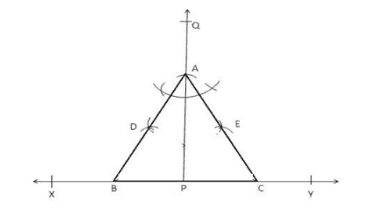Q.6: Construct a triangle ABC in which BC = 5 cm, AB = 38 cm and AC = 26 cm.

Sol:

Steps of construction:

(i) Draw a line segment BC= 5 cm.

(ii) With center B and radius equal to 3.8 cm draw an arc.

(iii) With center B and radius equal to 3.8 cm draw another arc which cuts the previously drawn arc at A.

(iv) Join AB and AC

Therefore, ABC$\bigtriangleup ABC$ is the required equilateral triangle.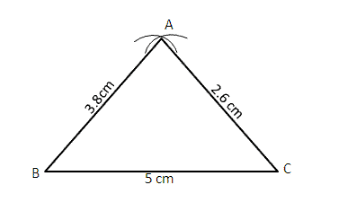Q.7: Construct a ΔABCinwhichBC=4.7cm,B=60andC=30$\Delta ABC in which BC = 4.7 cm, \angle B = 60^{\circ} and \angle C = 30^{\circ}$

Sol:

Steps of construction:

(i) Draw a line segment BC= 5cm.

(ii) At B draw XBC=60$\angle XBC=60^{\circ}$

(iii) At C draw YCB=30$\angle YCB =30^{\circ}$

Let XB and YC interact at A.

Therefore, ABC$\bigtriangleup ABC$ is the required triangle.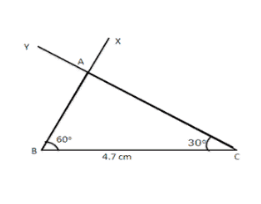Q.8: Construct an isosceles triangle PQR whose base measures 5 cm and each of equal sides measures 4.5 cm.

Sol:

Steps of construction:

(i) Draw a line segment QR= 5cm which is the base.

(ii) With center Q and radius equal to 4.5 cm, draw an arc.

(iii) With center P and the same radius is drawn another arc which cuts the previous arc at P.

(iv) Join PQ and PR.

Therefore, PQR$\bigtriangleup PQR$ is the required isosceles triangle.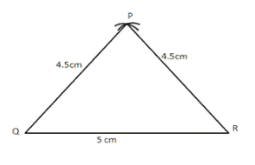Q.9: Construct an isosceles triangle whose base is 4.8 cm and whose vertical angle is 800

Sol:

Steps of construction:

(i) Draw a line segment BC = 4.8 cm

(ii) Make CBX=80$\angle CBX= 80^{\circ}$, below the line segment BC.

(iii) Make XBY=90$\angle XBY= 90^{\circ}$

(iv) Draw the right bisector PQ of BC, intersecting BY at O.

(v) With O as center and radius OB, draw a circle intersecting PQ at A.

(vi) Join AB and AC.

Therefore, PQR$\bigtriangleup PQR$ is the required isosceles triangle in which AB=AC.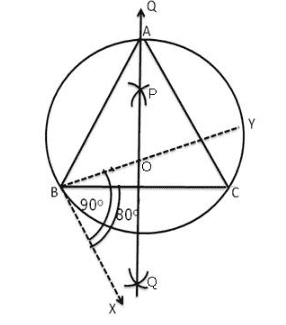Q.10: Construct a right-angled triangle whose hypotenuse measures 5.3 am and the length of one of whose sides containing the right angle measures 4.5 cm.

Sol:

Steps of construction:

(i) Draw a line segment BC= 5.3 cm.

(ii) Find the mid-point O of BC.

(iii) With O as a center and radius OB, draw a semicircle on BC.

(iv) With B as center and radius equal to 4.5 cm draw an arc cutting the semicircle at A.

(v) Join AB and AC.

Therefore, ABC$\bigtriangleup ABC$ is the required triangle.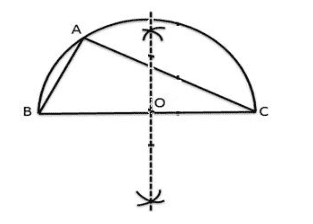Q.11: Construct a ΔABC$\Delta ABC$ in which B=30,B=60$\angle B = 30^{\circ} , \angle B = 60^{\circ}$ and the length of the perpendicular form the vertex A to the base BC is 4.8 cm.

Sol:

Steps of construction:

(i) Draw a line segment XY.

(ii) Take any point P on XY and draw PQXY$PQ\perp XY$.

(iii) Along PQ, set off PA= 4.8 cm.

(iv) Through A, draw LMXY$LM\parallel XY$.

(v) Construct LAB=30$\angle LAB= 30^{\circ}$ and MAC=60$\angle MAC= 60^{\circ}$ meeting XY at B and C respectively.

Therefore, ABC$\bigtriangleup ABC$ is the required triangle.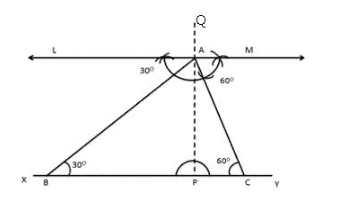Q12: Construct a triangle PQR whose perimeter is 12 am and lengths of whose sides are in the ratio 3:2:4.

Sol:

Steps of construction:

(i) Draw a line segment AB = 12 cm.

(ii) Draw a ray AX, making an acute angle with AB and draw in the downward direction.

(iii) From A set of (3+2+4) = 9 equal distances along AX.

(iv) Mark points L,M,N on AX such as that AL = 3 units, Lm = 2 units and MN = 4 units.

(v) Join NB.

(vi) Through L and M, draw LQNBandMRNB$LQ\parallel NB \; and\; MR\parallel NB$ cutting AB at Q and R respectively.

(vii) With Q as center and radius AQ, draw an arc.

(viii) With R as center and radius RB, draw another arc, cutting the previous arc at P.

(ix) Join PQ and PR.

Therefore, PQR$\bigtriangleup PQR$ is the required triangle.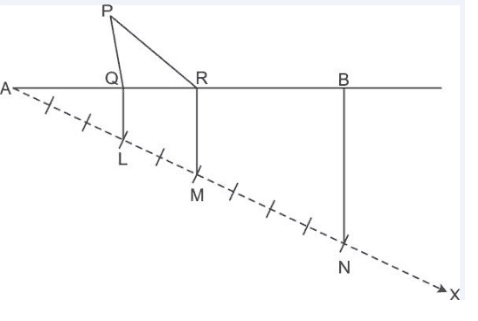Q13: Construct a ΔABC$\Delta ABC$ in which BC = 4.5 cm , B=60$\angle B = 60^{\circ}$ and the sum of the other two sides is 8 cm.

Sol:

Steps of Construction:

(i) Draw BC = 4.5 cm

(ii) Construct CBX=60$\angle CBX=60^{\circ}$

(iii) Along BX set off BP = 8 cm

(iv) Join CP.

(v) Draw the perpendicular bisector of CP to intersecting BP at A.

(vi) Join AC.

Therefore, ABC$\bigtriangleup ABC$ is the required triangle.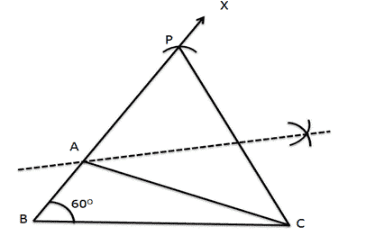Q14: Construct a ΔABC$\Delta ABC$ in which BC = 5.2 cm, B=30$\angle B = 30^{\circ}$ and the difference of the other two sides is 3.5 cm.

Sol:

Steps of Construction:

(i) Draw BC= 5.2 cm

(ii) Construct CBX=30$\angle CBX= 30^{\circ}$

(iii) Along BX set off BP = 3.5 cm

(iv) Join PC.

(v) Draw the perpendicular bisector of PC meeting BP produced at A.

(vi) Join AC.

Therefore, ABC$\bigtriangleup ABC$ is the required triangle.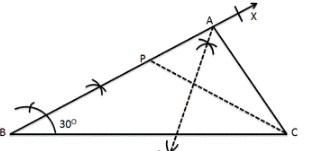### RS Aggarwal Class 9 Solutions Chapter 12 – Geometrical Constructions

Students can improve their mathematical skills by practicing as many as questions they can. And, for this purpose, referring to the RS Aggarwal Maths Solutions will help them to enhance their skills and improve question-solving time. The RS Aggarwal maths textbook gives them an opportunity to solve different types of complex questions. It helps in understanding the difficult concepts in easy way. Practicing this way students can master the subject over a period of time and will be able to score excellently in the exam.